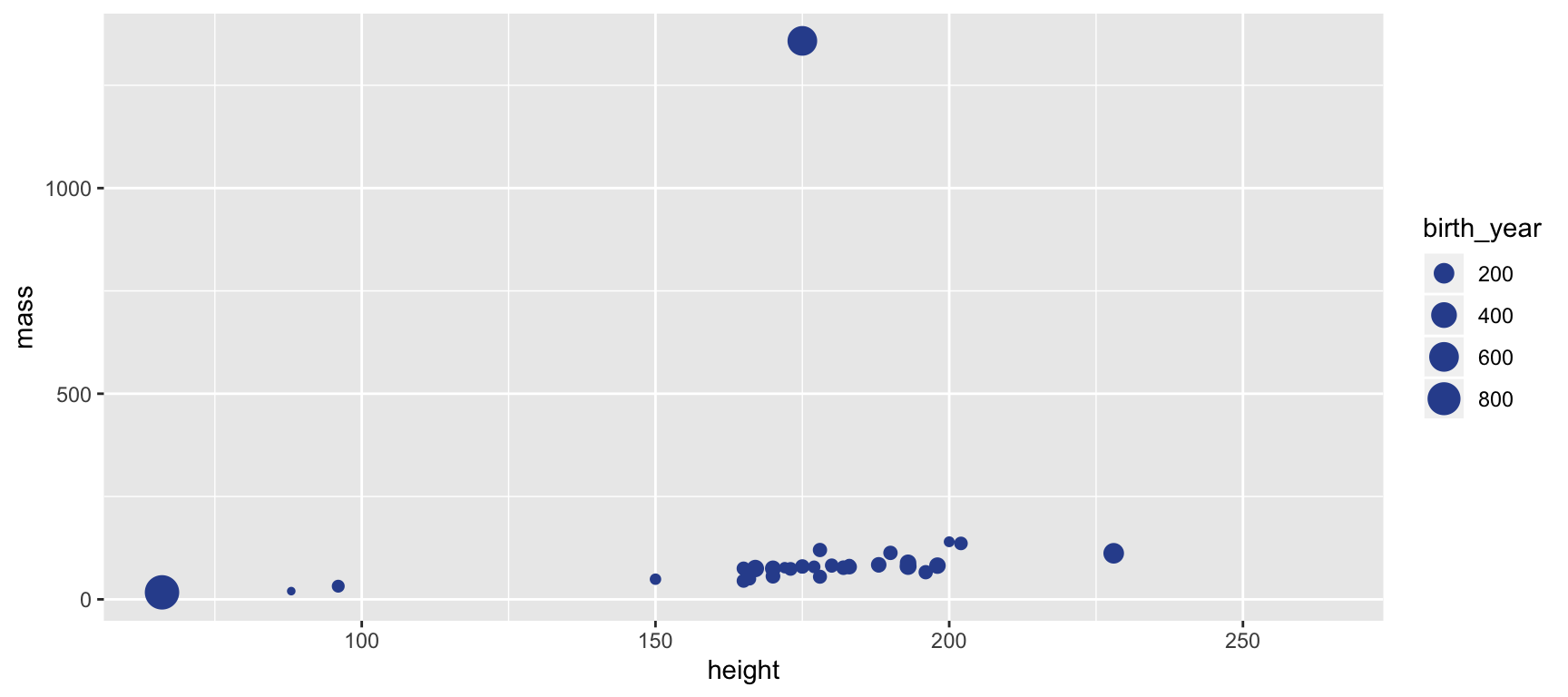### Modify the following plot to change the color of all points to "pink".

ggplot(data = starwars,
mapping = aes(x = height, y = mass, size = birth_year)) +
geom_point(color = "#30509C")
## Warning: Removed 51 rows containing missing values (geom_point).### Modify the following code to create a boxplot of height grouped by gender

After editing the code, change the chunk option to eval = TRUE.

ggplot(data = starwars,
mapping = aes(x = gender, y = height)) +
geom_---()